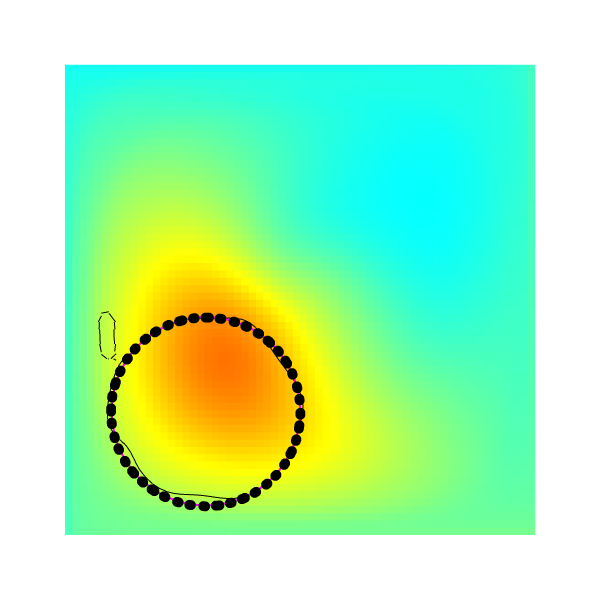Diffusive tracer, vof tracer and particle tracers.

The case is based on a test case for the vof advection. Results are visual:

Swirling tracersThe Magenta circle is the exact solution

#include  //diffusive tracer field
#include "vof.h"       //Interface tracer
#include "view.h"
#define BVIEW 1
#include  //Particles

scalar f[], s[], * interfaces = {f}, * tracers = {s};

double T = 15;
int main () {
L0 = 1;
X0 = Y0 = -L0/2;
DT = 0.1;
N = 64;
P_RK3 = true; // <- this improved the result considerably
run();
}

#define circle(x,y) (sq(0.2) - (sq(x + 0.2) + sq(y + .236338)))

event init (t = 0) {
fraction (f, circle(x,y));   // 1 line for initialization
foreach()
s[] = exp(10*circle(x,y)); // 2 lines

n_part = 100;
loc = malloc (sizeof(coord)*n_part);  //5 lines
foreach_particle() {
p_x() = -0.2 + 0.2*sin((double)j);
p_y() = -0.236338 + 0.2*cos((double)j);
}
}

event velocity (i++) {
vertex scalar psi[];
foreach_vertex()
psi[] = - 1.5*sin(2.*pi*t/T)*sin((x + 0.5)*pi)*sin((y + 0.5)*pi)/pi;
trash ({u});
struct { double x, y; } f = {-1.,1.};
foreach_face()
u.x[] = f.x*(psi[0,1] - psi[])/Delta;
boundary ((scalar *){u});
}

event movie (t += 0.1) {
draw_vof("f");
squares ("s");
scatter (loc);
save ("mov.mp4");
}

event stop (t = T) {
scalar b[];
fraction (b, circle(x,y));
draw_vof("b", lc = {1,0,1}, lw = 2);
draw_vof("f");
squares ("s");
translate (z = 0.01)
scatter (loc_n);
save ("result.png");
}